Courses

# Series I, Non Verbal Reasoning

## 25 Questions MCQ Test General Intelligence and Reasoning for SSC CGL | Series I, Non Verbal Reasoning

Description
This mock test of Series I, Non Verbal Reasoning for LR helps you for every LR entrance exam. This contains 25 Multiple Choice Questions for LR Series I, Non Verbal Reasoning (mcq) to study with solutions a complete question bank. The solved questions answers in this Series I, Non Verbal Reasoning quiz give you a good mix of easy questions and tough questions. LR students definitely take this Series I, Non Verbal Reasoning exercise for a better result in the exam. You can find other Series I, Non Verbal Reasoning extra questions, long questions & short questions for LR on EduRev as well by searching above.
QUESTION: 1

### Directions to Solve Each of the following questions consists of five figures marked A, B, C, D and E called the Problem Figures followed by five other figures marked 1, 2, 3, 4 and 5 called the Answer Figures. Select a figure from amongst the Answer Figures which will continue the same series as established by the five Problem Figures. Question - Select a figure from amongst the Answer Figures which will continue the same series as established by the five Problem Figures.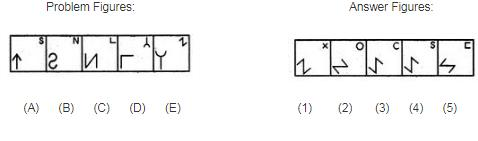Solution:

In each step, element at the upper-right position gets enlarged, inverts vertically and reaches the lower-left corner; the existing element at the lower-left position, is lost and a new small element appears at the upper-right position.

QUESTION: 2

### Directions to Solve Each of the following questions consists of five figures marked A, B, C, D and E called the Problem Figures followed by five other figures marked 1, 2, 3, 4 and 5 called the Answer Figures. Select a figure from amongst the Answer Figures which will continue the same series as established by the five Problem Figures. Question - Select a figure from amongst the Answer Figures which will continue the same series as established by the five Problem Figures.Problem Figures:                          Answer Figures: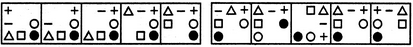(A)     (B)     (C)     (D)     (E)       (1)     (2)     (3)     (4)     (5)

Solution: In each step, the CW-end element moves to the ACW-end position.
QUESTION: 3

### Directions to Solve Each of the following questions consists of five figures marked A, B, C, D and E called the Problem Figures followed by five other figures marked 1, 2, 3, 4 and 5 called the Answer Figures. Select a figure from amongst the Answer Figures which will continue the same series as established by the five Problem Figures. Question - Select a figure from amongst the Answer Figures which will continue the same series as established by the five Problem Figures. Problem Figures:                          Answer Figures: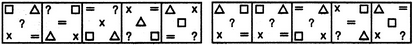(A)     (B)     (C)     (D)     (E)       (1)     (2)     (3)     (4)     (5)

Solution:

In each step, the elements move in the sequence.

QUESTION: 4

Directions to Solve

Each of the following questions consists of five figures marked A, B, C, D and E called the Problem Figures followed by five other figures marked 1, 2, 3, 4 and 5 called the Answer Figures. Select a figure from amongst the Answer Figures which will continue the same series as established by the five Problem Figures.

Question -

Select a figure from amongst the Answer Figures which will continue the same series as established by the five Problem Figures.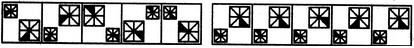(A)     (B)     (C)     (D)     (E)       (1)     (2)     (3)     (4)     (5)

Solution:

Both the larger and the smaller squares move to the adjacent corner ACW in each turn. Also, the shading in the smaller square moves 1, 2, 3, 4, 5, ... steps ACW sequentially and the shading in the larger square moves 1, 2, 3, 4, 5,.... steps CW sequentially.

QUESTION: 5
Directions to Solve

Each of the following questions consists of five figures marked A, B, C, D and E called the Problem Figures followed by five other figures marked 1, 2, 3, 4 and 5 called the Answer Figures. Select a figure from amongst the Answer Figures which will continue the same series as established by the five Problem Figures.

Question -

Select a figure from amongst the Answer Figures which will continue the same series as established by the five Problem Figures.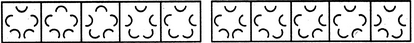(A)     (B)     (C)     (D)     (E)       (1)     (2)     (3)     (4)     (5)
Solution: One, two, three, one, two, three.....arcs get inverted sequentially. This inversion takes place in an ACW direction.
QUESTION: 6
Directions to Solve

Each of the following questions consists of five figures marked A, B, C, D and E called the Problem Figures followed by five other figures marked 1, 2, 3, 4 and 5 called the Answer Figures. Select a figure from amongst the Answer Figures which will continue the same series as established by the five Problem Figures.

Question -

Select a figure from amongst the Answer Figures which will continue the same series as established by the five Problem Figures.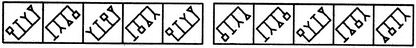(A)     (B)     (C)     (D)     (E)       (1)     (2)     (3)     (4)     (5)
Solution: Similar figure reappears in every second step. Each time the first figure reappears, the elements interchange positions in the order :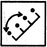. And, each time the second figure reappears, the elements interchange positions in the order :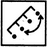QUESTION: 7
Directions to Solve

Each of the following questions consists of five figures marked A, B, C, D and E called the Problem Figures followed by five other figures marked 1, 2, 3, 4 and 5 called the Answer Figures. Select a figure from amongst the Answer Figures which will continue the same series as established by the five Problem Figures.

Question -

Select a figure from amongst the Answer Figures which will continue the same series as established by the five Problem Figures.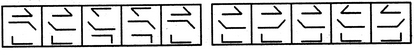(A)     (B)     (C)     (D)     (E)       (1)     (2)     (3)     (4)     (5)
Solution: In One step, the middle element rotates through 180o and in the next step, the other two elements rotate through 180o. The two steps are repeated alternately.
QUESTION: 8
Directions to Solve

Each of the following questions consists of five figures marked A, B, C, D and E called the Problem Figures followed by five other figures marked 1, 2, 3, 4 and 5 called the Answer Figures. Select a figure from amongst the Answer Figures which will continue the same series as established by the five Problem Figures.

Question -

Select a figure from amongst the Answer Figures which will continue the same series as established by the five Problem Figures.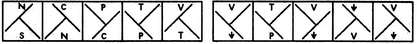(A)     (B)     (C)     (D)     (E)       (1)     (2)     (3)     (4)     (5)
Solution: The 'T' shaped large element rotates 90oCW in each step. The two small symbols interchange positions and the symbol that reaches the upper position gets replaced by a new one.
QUESTION: 9
Directions to Solve

Each of the following questions consists of five figures marked A, B, C, D and E called the Problem Figures followed by five other figures marked 1, 2, 3, 4 and 5 called the Answer Figures. Select a figure from amongst the Answer Figures which will continue the same series as established by the five Problem Figures.

Question -

Select a figure from amongst the Answer Figures which will continue the same series as established by the five Problem Figures.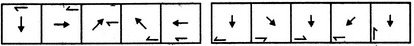(A)     (B)     (C)     (D)     (E)       (1)     (2)     (3)     (4)     (5)
Solution: The central arrow rotates 90oACW and 45oCW alternately. The half-arrow moves half-a-side of the square boundary in a CW direction and its head turns to the other side of the line in each step.
QUESTION: 10
Directions to Solve

Each of the following questions consists of five figures marked A, B, C, D and E called the Problem Figures followed by five other figures marked 1, 2, 3, 4 and 5 called the Answer Figures. Select a figure from amongst the Answer Figures which will continue the same series as established by the five Problem Figures.

Question -

Select a figure from amongst the Answer Figures which will continue the same series as established by the five Problem Figures.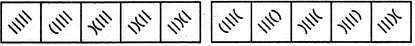(A)     (B)     (C)     (D)     (E)       (1)     (2)     (3)     (4)     (5)
Solution: In the first step, the lowermost line segment is converted into a curve. In the second step, the second line segment also gets converted into a curve and the (existing curve is inverted. In each subsequent step, all the elements (line segments and curves) move in the sequence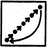QUESTION: 11
Directions to Solve

Each of the following questions consists of five figures marked A, B, C, D and E called the Problem Figures followed by five other figures marked 1, 2, 3, 4 and 5 called the Answer Figures. Select a figure from amongst the Answer Figures which will continue the same series as established by the five Problem Figures.

Question -

Select a figure from amongst the Answer Figures which will continue the same series as established by the five Problem Figures.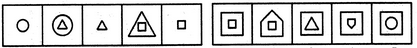(A)     (B)     (C)     (D)     (E)       (1)     (2)     (3)     (4)     (5)
Solution: In one step, the existing element enlarges and a new element appears inside this element. In the next step, the outer element is lost.
QUESTION: 12
Directions to Solve

Each of the following questions consists of five figures marked A, B, C, D and E called the Problem Figures followed by five other figures marked 1, 2, 3, 4 and 5 called the Answer Figures. Select a figure from amongst the Answer Figures which will continue the same series as established by the five Problem Figures.

Question -

Select a figure from amongst the Answer Figures which will continue the same series as established by the five Problem Figures.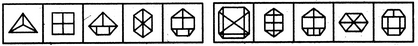(A)     (B)     (C)     (D)     (E)       (1)     (2)     (3)     (4)     (5)
Solution: The number of parts increases by one along with the number of sides in the figure.
QUESTION: 13
Directions to Solve

Each of the following questions consists of five figures marked A, B, C, D and E called the Problem Figures followed by five other figures marked 1, 2, 3, 4 and 5 called the Answer Figures. Select a figure from amongst the Answer Figures which will continue the same series as established by the five Problem Figures.

Question -

Select a figure from amongst the Answer Figures which will continue the same series as established by the five Problem Figures.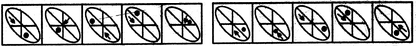(A)     (B)     (C)     (D)     (E)       (1)     (2)     (3)     (4)     (5)
Solution: In each step, the dot moves one space CW and the arrow moves two spaces CW.
QUESTION: 14
Directions to Solve

Each of the following questions consists of five figures marked A, B, C, D and E called the Problem Figures followed by five other figures marked 1, 2, 3, 4 and 5 called the Answer Figures. Select a figure from amongst the Answer Figures which will continue the same series as established by the five Problem Figures.

Question -

Select a figure from amongst the Answer Figures which will continue the same series as established by the five Problem Figures.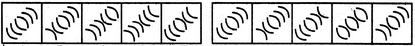(A)     (B)     (C)     (D)     (E)       (1)     (2)     (3)     (4)     (5)
Solution: One arc and four arcs get inverted alternately.
QUESTION: 15
Directions to Solve

Each of the following questions consists of five figures marked A, B, C, D and E called the Problem Figures followed by five other figures marked 1, 2, 3, 4 and 5 called the Answer Figures. Select a figure from amongst the Answer Figures which will continue the same series as established by the five Problem Figures.

Question -

Select a figure from amongst the Answer Figures which will continue the same series as established by the five Problem Figures.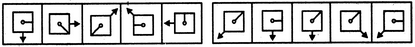(A)     (B)     (C)     (D)     (E)       (1)     (2)     (3)     (4)     (5)
Solution: The pin rotates 45oCW and 90oCW alternately and moves one space (each space is equal to half-a-side of the square) and two spaces CW alternately. The arrow rotates 90oACW and 45oACW alternately and moves two spaces and one space.
QUESTION: 16
Directions to Solve

Each of the following questions consists of five figures marked A, B, C, D and E called the Problem Figures followed by five other figures marked 1, 2, 3, 4 and 5 called the Answer Figures. Select a figure from amongst the Answer Figures which will continue the same series as established by the five Problem Figures.

Question -

Select a figure from amongst the Answer Figures which will continue the same series as established by the five Problem Figures.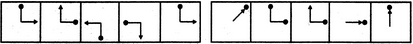(A)     (B)     (C)     (D)     (E)       (1)     (2)     (3)     (4)     (5)
Solution: In each step, the pin rotates 90oCW and the arrow rotates 90oACW.
QUESTION: 17
Directions to Solve

Each of the following questions consists of five figures marked A, B, C, D and E called the Problem Figures followed by five other figures marked 1, 2, 3, 4 and 5 called the Answer Figures. Select a figure from amongst the Answer Figures which will continue the same series as established by the five Problem Figures.

Question -

Select a figure from amongst the Answer Figures which will continue the same series as established by the five Problem Figures.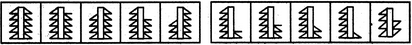(A)     (B)     (C)     (D)     (E)       (1)     (2)     (3)     (4)     (5)
Solution: In one step, the figure gets laterally inverted and one line segment is lost from the upper end of the RHS portion of the figure. In the next step, the figure gets laterally inverted and one line segment is lost from the upper end of the LHS portion of the figure.
QUESTION: 18
Directions to Solve

Each of the following questions consists of five figures marked A, B, C, D and E called the Problem Figures followed by five other figures marked 1, 2, 3, 4 and 5 called the Answer Figures. Select a figure from amongst the Answer Figures which will continue the same series as established by the five Problem Figures.

Question -

Select a figure from amongst the Answer Figures which will continue the same series as established by the five Problem Figures.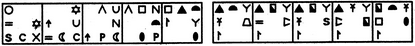(A)     (B)     (C)     (D)     (E)       (1)     (2)     (3)     (4)     (5)
Solution: All the elements move half-a-side of the square boundary in ACW direction in each step. Also, first, third and fifth elements are replaced by new elements in one step and second, fourth and sixth elements are replaced by new elements in the next step. The two steps are repeated alternately.
QUESTION: 19
Directions to Solve

Each of the following questions consists of five figures marked A, B, C, D and E called the Problem Figures followed by five other figures marked 1, 2, 3, 4 and 5 called the Answer Figures. Select a figure from amongst the Answer Figures which will continue the same series as established by the five Problem Figures.

Question -

Select a figure from amongst the Answer Figures which will continue the same series as established by the five Problem Figures.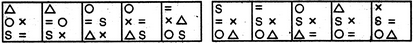(A)     (B)     (C)     (D)     (E)       (1)     (2)     (3)     (4)     (5)
Solution: The elements move in the sequence's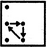and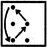alternately.
QUESTION: 20
Directions to Solve

Each of the following questions consists of five figures marked A, B, C, D and E called the Problem Figures followed by five other figures marked 1, 2, 3, 4 and 5 called the Answer Figures. Select a figure from amongst the Answer Figures which will continue the same series as established by the five Problem Figures.

Question -

Select a figure from amongst the Answer Figures which will continue the same series as established by the five Problem Figures.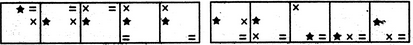(A)     (B)     (C)     (D)     (E)       (1)     (2)     (3)     (4)     (5)
Solution: In the first step, the ACW end element moves two spaces (each space is equal to half-a-side of the square boundary) in an ACW direction. In the second step, the CW-end element moves three spaces ACW. In the third step, the remaining element moves four spaces ACW. The three steps are repeated to continue the series.
QUESTION: 21
Directions to Solve

Each of the following questions consists of five figures marked A, B, C, D and E called the Problem Figures followed by five other figures marked 1, 2, 3, 4 and 5 called the Answer Figures. Select a figure from amongst the Answer Figures which will continue the same series as established by the five Problem Figures.

Question -

Select a figure from amongst the Answer Figures which will continue the same series as established by the five Problem Figures.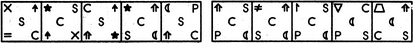(A)     (B)     (C)     (D)     (E)       (1)     (2)     (3)     (4)     (5)
Solution: The symbols move in the sequence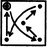in the first step. In each subsequent step, the symbols move in the sequence obtained by rotating the previous sequence through 90oACW. Also, in each step, the symbol that reaches the encircled position gets replaced by a new one.
QUESTION: 22
Directions to Solve

Each of the following questions consists of five figures marked A, B, C, D and E called the Problem Figures followed by five other figures marked 1, 2, 3, 4 and 5 called the Answer Figures. Select a figure from amongst the Answer Figures which will continue the same series as established by the five Problem Figures.

Question -

Select a figure from amongst the Answer Figures which will continue the same series as established by the five Problem Figures.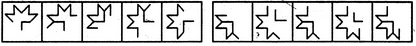(A)     (B)     (C)     (D)     (E)       (1)     (2)     (3)     (4)     (5)
Solution: In each step, one line segment is lost from the CW-end of the outer element and a new line segment appears at the ACW-end. Also, the inner 'L' shaped element rotates 90oCW in each step.
QUESTION: 23
Directions to Solve

Each of the following questions consists of five figures marked A, B, C, D and E called the Problem Figures followed by five other figures marked 1, 2, 3, 4 and 5 called the Answer Figures. Select a figure from amongst the Answer Figures which will continue the same series as established by the five Problem Figures.

Question -

Select a figure from amongst the Answer Figures which will continue the same series as established by the five Problem Figures.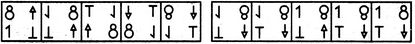(A)     (B)     (C)     (D)     (E)       (1)     (2)     (3)     (4)     (5)
Solution: In each step, all the elements move to the adjacent corner (of the square boundary) in a CW direction and the element that reaches the upper-left corner gets vertically inverted.
QUESTION: 24
Directions to Solve

Each of the following questions consists of five figures marked A, B, C, D and E called the Problem Figures followed by five other figures marked 1, 2, 3, 4 and 5 called the Answer Figures. Select a figure from amongst the Answer Figures which will continue the same series as established by the five Problem Figures.

Question -

Select a figure from amongst the Answer Figures which will continue the same series as established by the five Problem Figures.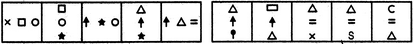(A)     (B)     (C)     (D)     (E)       (1)     (2)     (3)     (4)     (5)
Solution: In each step, the first element moves to the third position and gets replaced by a new element; the second and the third elements move to the first and the second positions respectively and the entire figure rotates 90oCW.
QUESTION: 25
Directions to Solve

Each of the following questions consists of five figures marked A, B, C, D and E called the Problem Figures followed by five other figures marked 1, 2, 3, 4 and 5 called the Answer Figures. Select a figure from amongst the Answer Figures which will continue the same series as established by the five Problem Figures.

Question -

Select a figure from amongst the Answer Figures which will continue the same series as established by the five Problem Figures.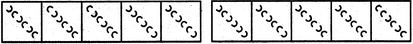Solution: We can label the arcs as shown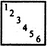. The arcs get inverted in the sequence (1 & 2), (3, 4 & 5), (6 & 1), (2, 3 & 4), (5 & 6),...........